# 802 Pi Day at Smith’s

In the United States tomorrow’s date is written 3-14. Because 3.14 is a famous approximation for π (pi), people all over the country will eat pie to celebrate Pi Day. This afternoon I took a picture of this sign and the pie display at my local Smith’s Food and Drug.I took that picture right when I walked into the store, but there were no pies on display for National Pi Day.About 15 minutes later I returned to the display to take another picture. Now there were pies on the table! I told a salesperson who I think worked on the display that I was going to take a picture and put it on my blog. She asked what kind of a blog I wrote. I told her a math blog. She looked puzzled and asked why I would want to put a picture of pies on a math blog. Then she turned around, looked at the display, and said something like, “Oh, now I get it, the number pi.”

How do you choose between apple, cherry, or peach pie? It’s much easier if you choose two and then you can get a free 8 oz. Cool Whip, too. Yummy.

If by chance you prefer pizza pi, here’s a thought from twitter that is often repeated in March:

And here’s some original artwork that displays pi in a way I had never thought of before:

BREAKING: secret of Pi revealed #PiDay pic.twitter.com/Ao8BQp31jd

You can also look here for a million digits of pi.

But pi is not the only interesting number in the world. Every number has its own curiosities. Let me tell you some reasons to get excited about the number 802:

802 is the sum of two squares:

• 21² + 19² = 802

So 802 is the hypotenuse of a Pythagorean triple:

• 80-798-802, which is 2 times another triple: 40-399-401.

It also means something else: Since odd numbers 21 and 19 have no common prime factors, 802 can be evenly divide by 2. Duh. . ., but it also means that unless 802 is also divisible by 5, 13, or 17, its only factors will be 2 and a prime number! Why are those three numbers the only ones I care about? Because they are the only prime number Pythagorean triple hypotenuses less than √802 ≈ 28.3.

Guess what? 5, 13, and 17 do not divide evenly into 802, so 802 is the product of 2 and a prime number which happens to be 401.

• 802 is a composite number.
• Prime factorization: 802 = 2 x 401
• The exponents in the prime factorization are 1 and 1. Adding one to each exponent and multiplying we get (1 + 1)(1 + 1) = 2 x 2 = 4. Therefore 802 has exactly 4 factors.
• Factors of 802: 1, 2, 401, 802
• Factor pairs: 802 = 1 x 802 or 2 x 401
• 802 has no square factors that allow its square root to be simplified. √802 ≈ 28.3196045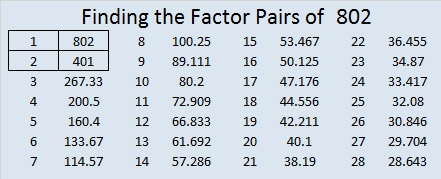Today’s puzzle is number 802 to distinguish it from every other puzzle I’ve made. Writing the numbers 1 – 10 in both the top row and the first column so that the factors and the clues work together as a multiplication table is as easy as pie!Print the puzzles or type the solution on this excel file: 10-factors 801-806

And here is a little more about the number 802:

802 is the sum of 8 consecutive prime numbers:

• 83 + 89 + 97 + 101 + 103 + 107 + 109 + 113 = 802

802 can also be written as the sum of three squares three different ways:

• 28² + 3² + 3² = 802
• 27² + 8² + 3² = 802
• 24² + 15² + 1² = 802

802 is also a palindrome in two other bases:

• 414 BASE 14 because 4(196) + 1(14) + 4(1) = 802
• 202 BASE 20 because 2(400) + 0(20) + 2(1) = 802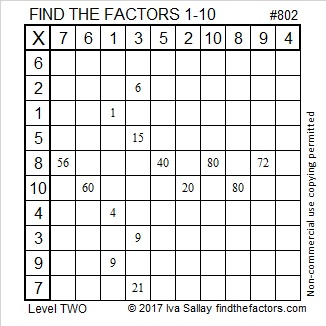# 628, Tau, Pi, and Level 6

The circumference of a circle with a radius of one is approximately 6.28. That’s an important enough number that it has been given the symbol “τ ” which is pronounced “tau”. τ looks a little like half of the number π, but τ = 2π.

Some people think we should get rid of π and only use τ. Other people feel that π has been used for centuries, and there is no compelling reason to change now.

π is perfect for finding the area of a circle: Area = πr². Here’s the area of a circle using tau: Area = r²τ/2.

τ is very good for finding the circumference of a circle: Circumference = τr, but that looks strange compared to 2πr. In fact, it can be difficult to tell if τr is one character or two.

The Tau Manifesto shows angle measurements in degrees, π radians and τ radians. You might want to look at some videos, too. Some people think the τ radians are simpler because the radians correspond exactly to the fractional pieces of the circumference of a circle or, get this, to the fractional pieces of a pie. (τ does that, not π.) Other people think that π radians are just as good because we’re used to them, and they correspond exactly to the area of any wedge in a unit circle or the area of any slice of pie. (Which would you rather eat the circumference or the area of a pie?)

Until I wrote this post and read the link shared in the comments, I hadn’t heard anybody say that π is better for some situations while τ is better for others. (Actually, it appears that π is better except in formulas that use 2π.) Diameters and radii have co-existed peacefully for centuries. I don’t understand why π and τ can’t do the same. Here’s a great video that shows both sides of the argument.

—————————————————————————————————

22² + 12² = 628.

628 is the hypotenuse of the Pythagorean triple 340-528-628. The greatest common factor of those three numbers is the same as the greatest common factor of 22² and 12².

7² + 11² + 13² + 17² = 628. Thank you OEIS.org for that fun fact about the squares of those four consecutive prime numbers.Print the puzzles or type the solution on this excel file: 12 Factors 2015-09-21

—————————————————————————————————

• 628 is a composite number.
• Prime factorization: 628 = 2 x 2 x 157, which can be written 628 = (2^2) x 157
• The exponents in the prime factorization are 2 and 1. Adding one to each and multiplying we get (2 + 1)(1 + 1) = 3 x 2  = 6. Therefore 628 has exactly 6 factors.
• Factors of 628: 1, 2, 4, 157, 314, 628
• Factor pairs: 628 = 1 x 628, 2 x 314, or 4 x 157
• Taking the factor pair with the largest square number factor, we get √628 = (√4)(√157) = 2√157 ≈ 25.059928.—————————————————————————————————# 425 and Level 6

425 ends in 25 so it can be divided evenly by 25. If I had \$4.25 all in quarters. How many quarters would I have? That’s the problem that I think of when I divide by 25. All of the factors of 425 are listed below the puzzle.

For some reason unknown to me, here in the United States, dates are ordered by month, date, and year. This rather illogical way of ordering allows us to say that today is 3-14-15, which are the first five digits of pi.  It could also be said that 3-14-15 at 9:26:53 gives the first ten digits of pi.

Logical or not, it is fun to declare today as Pi Day. Today’s puzzle celebrates those first five digits:Print the puzzles or type the factors on this excel file: 12 Factors 2015-03-09

• 425 is a composite number.
• Prime factorization: 425 = 5 x 5 x 17, which can be written 425 = (5^2) x 17
• The exponents in the prime factorization are 2 and 1. Adding one to each and multiplying we get (2 + 1)(1 + 1) = 3 x 2  = 6. Therefore 425 has exactly 6 factors.
• Factors of 425: 1, 5, 17, 25, 85, 425
• Factor pairs: 425 = 1 x 425, 5 x 85, or 17 x 25
• Taking the factor pair with the largest square number factor, we get √425 = (√25)(√17) = 5√17 ≈ 20.6155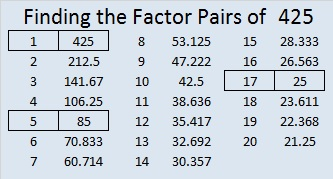425 and all of it factors (except 1) are hypotenuses of primitive Pythagorean triples, so 425 is the hypotenuse of several triples:

• [87-416-425] and
• [297-304-425] are primitives
• [65-420-425] is [13-84-85] times 5
• [119-408-425] is [7-24-25] times 17
• [180-385-425] is [36-77-85] times 5
• [200-375-425] is [8-15-17] times 25
• [255-340-425] is [3-4-5] times 85# 314 and The Pi Day of Our Lives

• 314 is a composite number.
• Prime factorization: 314 = 2 x 157
• The exponents in the prime factorization are 1 and 1. Adding one to each and multiplying we get (1 + 1)(1 + 1) = 2 x 2 = 4. Therefore 314 has exactly 4 factors.
• Factors of 314: 1, 2, 157, 314
• Factor pairs: 314 = 1 x 314 or 2 x 157
• 314 has no square factors that allow its square root to be simplified. √314 ≈ 17.720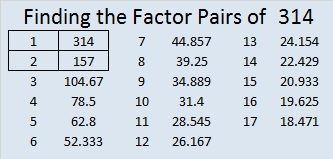On 3-14-15 at 9:26:53 we will experience the most significant pi day of our lives.

is selling wristbands, t-shirts, and baseball caps commemorating this special upcoming event at very reasonable prices.Each wristband costs only \$3.14 (minimum purchase is 3 wristbands) while the t-shirts and the baseball caps are only \$20 each. The website offers free shipping on everything they offer anywhere! You might just want to add one or more of these items to your Christmas wishlist!

# 30 and Pieces of Pi

### Pieces of Pi:Haha. There’s no easy way to cut a pie into seven equal slices. . .

But you can find beautiful representations of π at Paula Krieg’s blog. It is a bookbinding blog with just the right artistic touch for pi expressed as π, a fraction (2 ways), or a decimal!

### Factors of 30:

30 is a composite number. 30 = 1 x 30, 2 x 15, 3 x 10, or 5 x 6. Factors of 30: 1, 2, 3, 5, 6, 10, 15, 30. Prime factorization: 30 = 2 x 3 x 5.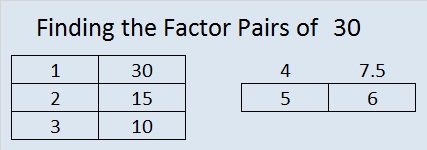When 30 is a clue in the FIND THE FACTORS puzzle, use 3 x 10 or 5 x 6. Only one of those pairs will work for that clue in that particular puzzle.

### Sum-Difference Puzzle:

30 has four factor pairs. One of those pairs adds up to 13, and another one subtracts to give us 13. If you can find those factor pairs, then you can solve this puzzle!